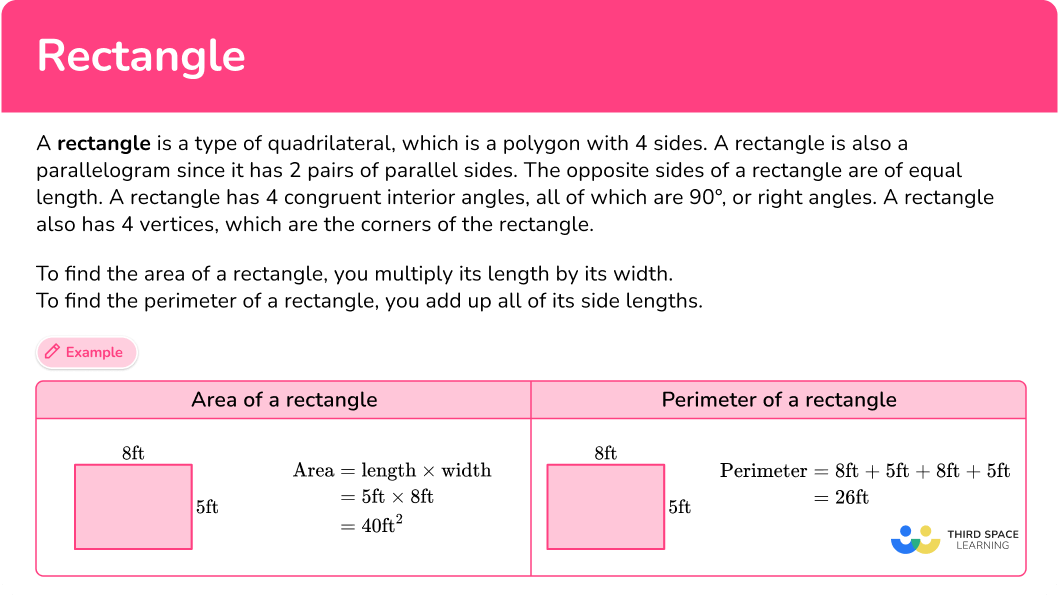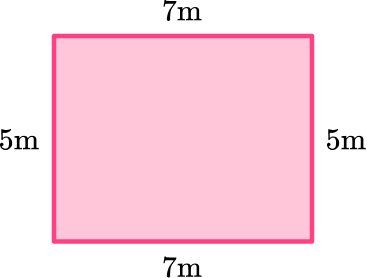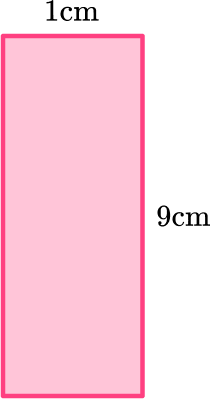# Rectangle

Here you will learn about rectangles, including how to identify a rectangle, the properties of a rectangle, and how to find the area of a rectangle and the perimeter of a rectangle.

Students will first learn about rectangles as part of geometry in 1 st grade and will expand upon their knowledge of rectangles throughout the elementary grades.

## What is a rectangle?

A rectangle is a type of quadrilateral, which is a polygon with 4 sides. The word rectangle comes from the Latin rectus (or rect) meaning “right” or “straight” and angulus meaning “angle.” A rectangle is also a parallelogram since it has 2 pairs of parallel sides.

The opposite sides of a rectangle are of equal length. A rectangle also has 4 congruent interior angles, all of which are 90^{\circ} , or right angles. A rectangle also has 4 vertices, which are the corners of the rectangle.

Beginning in 3 rd grade, students will learn to find the area and perimeter of a rectangle.

To find the area of a rectangle, you multiply its length by its width. To find the perimeter of a rectangle, you add up all of its side lengths.

For example,

Area of a rectanglePerimeter of a rectangle

\begin{aligned} \text { Area } & =\text { length } \times \text { width } \\ & =5 \mathrm{ft} \times 8 \mathrm{ft} \\ & =40 \mathrm{ft}^2 \end{aligned}

\begin{aligned} \text { Perimeter } & =8 \mathrm{ft}+5 \mathrm{ft}+8 \mathrm{ft}+5 \mathrm{ft} \\ & =26 \mathrm{ft} \end{aligned}

### What is a rectangle?## Common Core State Standards

How does this relate to 1 st grade math through 5 th grade math?

• Grade 1 – Geometry (1.G.A.1)
Distinguish between defining attributes (for example, triangles are closed and three-sided) versus non-defining attributes (for example, color, orientation, overall size); build and draw shapes to possess defining attributes.

• Grade 2 – Geometry (2.G.A.1)
Recognize and draw shapes having specified attributes, such as a given number of angles or a given number of equal faces. Identify triangles, quadrilaterals, pentagons, hexagons, and cubes.

• Grade 3 – Geometry (3.G.A.1)
Understand that shapes in different categories (for example, rhombuses, rectangles, and others) may share attributes (example, having four sides), and that the shared attributes can define a larger category (for example, quadrilaterals). Recognize rhombuses, rectangles, and squares as examples of quadrilaterals, and draw examples of quadrilaterals that do not belong to any of these subcategories.

• Grade 3 – Geometry (3.MD.D.8)
Solve real world and mathematical problems involving perimeters of polygons, including finding the perimeter given the side lengths, finding an unknown side length, and exhibiting rectangles with the same perimeter and different areas or with the same area and different perimeters.

• Grade 5 – Geometry (5.G.B.3)
Understand that attributes belonging to a category of two-dimensional figures also belong to all subcategories of that category. For example, all rectangles have four right angles and squares are rectangles, so all squares have four right angles.

## How to identify a rectangle

In order to identify a rectangle:

1. Look for the characteristics of a rectangle.
2. State whether or not the shape is a rectangle.
3. If the shape is not a rectangle, explain what characteristics are different.

## Rectangle examples

### Example 1: identify rectangles

Is this shape a rectangle?

1. Look for the characteristics of a rectangle.

A rectangle has 4 sides, 4 right angles, 2 pairs of parallel sides, and 2 pairs of opposite sides that are of equal length.

2State whether or not the shape is a rectangle.

This shape is a rectangle.

### Example 2: identify rectangles

Is this shape a rectangle?

A rectangle has 4 sides, 4 right angles, 2 pairs of parallel sides, and 2 pairs of opposite sides that are of equal length.

This shape is a rectangle. It is also a square since it has 4 equal sides.

### Example 3: identify rectangles

Is this shape a rectangle?

A rectangle has 4 sides, 4 right angles, 2 pairs of parallel sides, and 2 pairs of opposite sides that are of equal length.

This shape is not a rectangle.

This shape does not have 4 right angles.

### Example 4: classify a rectangle

Henry wants to classify the shape shown below. Which of the following names can this shape be classified as?

polygon, quadrilateral, parallelogram, rectangle, trapezoid, kite, rhombus, square

A rectangle has 4 sides, 4 right angles, 2 pairs of parallel sides, and 2 pairs of opposite sides that are of equal length.

This shape is a rectangle. It is also a polygon, quadrilateral, and a parallelogram.

The shape is not a trapezoid, kite, rhombus, or square.

## How to find the area of a rectangle

In order to find the area of a rectangle:

1. Determine the length and width of the rectangle.
2. Multiply length times width.
3. Label the area with square units.

### Example 5: area of a rectangle

Find the area of rectangle ABCD .

The length of the rectangle is 9 inches and the width of the rectangle is 4 inches.

\begin{aligned} \text {Area } & =\text { length } \times \text { width } \\\\ & =9 \times 4 \\\\ & =36 \end{aligned}

\text {Area }=36 \text { square inches }

## How to find the perimeter of a rectangle

In order to find the perimeter of a rectangle:

1. Determine the side lengths of the rectangle.
2. Add the side lengths together.
3. Label the perimeter with the correct units.

### Example 6: perimeter of a rectangle

Find the perimeter of the rectangle.

The side lengths are 10{~cm}, 10{~cm}, 3{~cm}, and 3{~cm} . Even though you are only given the length and width, you will use each of them twice since the opposite sides are the same length.

\begin{aligned} \text {Perimeter } & =10+10+3+3 \\\\ & =26 \end{aligned}

\text {Perimeter }= 26 \mathrm{~cm}

### Teaching tips for rectangles

• Show students how rectangles are present in their daily lives. Point out real-life rectangular shapes like windows, doors, book covers, and tables. Encourage students to identify rectangles in their surroundings.

• To help students understand classification, provide a variety of 2D shapes (hands-on shapes or worksheets on rectangles) and ask students to sort them into categories based on their properties. Include rectangles in the sorting activity and have students explain why certain shapes belong in the rectangle category.

### Easy mistakes to make

• Confusing sides and angles
A rectangle has four sides, and opposite sides are equal in length. However, students sometimes mistake the lengths of the sides for the measures of the angles. Remember that a rectangle has four right angles, each measuring 90 degrees.

• Assuming all rectangles are squares
A square is a special type of rectangle where all four sides are equal in length. However, not all rectangles are squares. Students might incorrectly assume that any shape with four sides is a square when it could be a rectangle with unequal side lengths.

• Incorrectly calculating the perimeter or area
The perimeter of a rectangle is calculated by adding the lengths of all four sides, while the area is calculated by multiplying the length and width. Students might forget to include all four sides or confuse the length and width when performing these calculations.

### Practice rectangle questions

1) Which shape is a rectangle?For a shape to be a rectangle, it must have 4 sides, 4 right angles, 2 pairs of parallel sides, and 2 pairs of opposite sides that are of equal length. The second shape is the only one with all of those properties.

2) Which shape is not a rectangle?For a shape to be a rectangle, it must have 4 sides, 4 right angles, 2 pairs of parallel sides, and 2 pairs of opposite sides that are of equal length.

The last shape is the only one without all of those properties since it does not have 4 right angles.

3) What is one property of a rectangle?

opposite sides are the same length4 equal sidesonly 1 pair of parallel sidesadjacent sides are always the same lengthThe properties of a rectangle are: 4 sides, 4 right angles, 2 pairs of parallel sides, and 2 pairs of opposite sides that are of equal length. Their adjacent sides may sometimes be the same length, such as in a square, but not always.

4) A rectangle is also a…

squaretrapezoidright triangleparallelogramA rectangle is also a parallelogram because in order for a shape to be a parallelogram, it must have 4 sides and 2 pairs of parallel sides. A rectangle has both of those properties, so it is a parallelogram.

5) What is the area of the rectangle?30{~m^2}24{~m^2}35{~m^2}42{~m^2}To find the area of a rectangle, you multiply the length of the rectangle by the width of the rectangle.

7 \mathrm{~m} \times 5 \mathrm{~m}=35 \mathrm{~m}^2

6) What is the perimeter of the rectangle?9{~cm}20{~cm}10{~cm}19{~cm}To find the perimeter of a rectangle, you need to add up its 4 sides. There are only 2 side lengths shown, but since you know that a rectangle has opposite sides that are the same length, you know the lengths of the non-labeled sides as well.

1 \mathrm{~cm}+1 \mathrm{~cm}+9 \mathrm{~cm}+9 \mathrm{~cm}=20 \mathrm{~cm}

## Rectangle FAQs

What is a rectangle?

A rectangle is a type of quadrilateral, which is a polygon with 4 sides, that has 2 pairs of parallel sides, 4 right angles, and opposite sides that are the same length.

What are the properties of a rectangle?

The properties of a rectangle are: 4 sides, 4 right angles, 2 pairs of parallel sides, and 2 pairs of opposite sides that are of equal length.

How do you find the area of a rectangle?

You can find the area of a rectangle by multiplying its length times its width.

Area of rectangle = length × width

How do you find the perimeter of a rectangle?

You can find the perimeter of a rectangle by adding up its 4 side lengths.

Perimeter of a rectangle = sum of all its 4 sides

How can you find the length of the diagonals of a rectangle?

The length can be calculated with the pythagorean theorem, if the other two dimensions of the rectangle are known.

## Still stuck?

At Third Space Learning, we specialize in helping teachers and school leaders to provide personalized math support for more of their students through high-quality, online one-on-one math tutoring delivered by subject experts.

Each week, our tutors support thousands of students who are at risk of not meeting their grade-level expectations, and help accelerate their progress and boost their confidence.